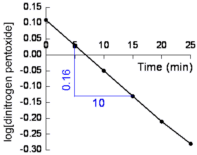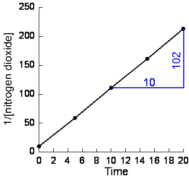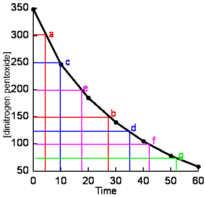Determining Order of Reaction and Rate Law Tutorial

Key Concepts

• The order with respect to one species in the reaction is the exponent on the concentration term for that species in the rate law.

For the rate law: rate = k[A][B]2

For Species: Exponent of
concentration
Rate is
A 1 first order
B 2 second order

• The order of a reaction is the sum of the exponents on the concentration terms in the rate law.

For the rate law: rate = k[A][B]2

order of reaction = exponent of [A] + exponent of [B]
order of reaction = 1 + 2 = 3
Reaction is third order.
• Several methods can be used to determine the rate law from experimental data.
The three most common methods are :
• (i) Several experiments are run at the same temperature, the rate at the start of each experiment is determined, then the results of all the experiments analyzed to determine the relationship between initial concentration and initial rate. Zero order reaction: rate is independent of the concentration of the species First order reaction: rate ∝ [species] Second order reaction: rate ∝ [species]2 First order reaction: A plot of log10[species] against time produces a straight line. The slope of the line = -k/2.303 Second order reaction: A plot of 1/[species] against time produces a straight line. The slope of the line = k Let: [A]0 = the initial concentration of species A k = specific rate constant for the reaction t = sometime later on in the experiment [A]t = the concentration of A at time t Then we find that there is an exponential relationship between the concentration of A and the length of time the experiment is allowed to run for: [A]t = [A]0 × e-kt So, by rearranging this equation we find that: [A]/[A]0 = e-kt Taking the natural logarithm (ln) of both sides of the equation we get: ln([A]/[A]0) = -kt or, by converting natural logarithms (ln) to logarithms to the base to (log10) we find that: log10[A] = -kt/2.303 + log[A]0 Half-life, t½, is the time taken for half the quantity of a reactant to be consumed, so log[A]½ = -kt½/2.303 + log[A]0 First order reaction: Half-life of a first order reaction is a constant, ie, half-life is independent of initial concentration. First order reaction: t½ = 0.693/k Second order reaction: Half-life of a second order reaction is not independent of intial concentration. For the second order reaction: A → B where rate = k[A]2 t½ = 1/k[A]

No ads = no money for us = no free stuff for you!

Examples of Initial Concentration Method to Determine Rate Law

Question 1 : Determining the Rate Law of a Zero Order Reaction

Ammonia, NH3(g), can be decomposed on the surface of tungsten metal to produce nitrogen, N2(g), and hydrogen, H2(g), according to the equation:

2NH3(g) → 3H2(g) + N2(g)

The results of a series of experiments carried out at the same temperature are shown below:

Trial Initial [NH3]
(M)
Initial Rate
(M/min)
1 2.50 × 10-3 6.67 × 10-7
2 5.00 × 10-3 6.67 × 10-7

Determine the rate law for this reaction.

Solution:

1. Determine the change in initial concentration:
The initial concentration of NH3 was increased by a factor of

 [NH3(trial 2)][NH3(trial 1)] = 5.00 × 10-32.50 × 10-3 = 2

2. Determine the change in initial rate as a result of this change in concentration:
The initial rate of the reaction increased by a factor of

 rate(trial 2)rate(trial 1) = 6.67 × 10-76.67 × 10-7 = 1

That is, the rate does NOT change when the concentration of NH3 changes.

3. Determine the relationship between the change in concentration and the change in rate:
Since increasing the concentration by a factor of 2 leads to no increase in the rate, the reaction rate is independent of the concentration of NH3

rate = k

The reaction is zero order.

4. Calculate the specific rate constant, k, for the reaction:

k = rate

Using data from Trial 1:

k = 6.67 × 10-7

5. Write the rate law for this reaction:

rate = 6.67 × 10-7

Question 2: Determining the Rate Law of a First Order Reaction

Azomethane, C2H6N2, decomposes to produce ethane, C2H6, and nitrogen, N2.

C2H6N2(g) → C2H6(g) + N2(g)

The results of a series of experiments carried out at the same temperature are shown below:

Trial Initial [C2H6N2(g)]
(M)
Initial Rate
(M/min)
1 2.00 × 10-2 3.00 × 10-4
2 4.00 × 10-2 6.00 × 10-4

Determine the rate law for this reaction.

Solution:

1. Determine the change in initial concentration:
The initial concentration of C2H6N2(g) was increased by a factor of

 [C2H6N2(g)(trial 2)][C2H6N2(g)(trial 1)] = 4.00 × 10-2 2.00 × 10-2 = 2

2. Determine the change in initial rate as a result of this change in concentration:
The initial rate of the reaction increased by a factor of

 rate(trial 2)rate(trial 1) = 6.00 × 10-43.00 × 10-4 = 2

3. Determine the relationship between the change in concentration and the change in rate:
Since increasing the concentration by a factor of 2 leads to an increase in the rate by a factor of 2, the reaction rate and the concentration of C2H6N2(g) are directly proportional:

rate ∝ [C2H6N2(g)]

So, rate = k[C2H6N2(g)]

The reaction is first order.

4. Calculate the specific rate constant, k, for the reaction:

 k = rate     [C2H6N2(g)]

Using data from Trial 1:

 k = 3.00 × 10-4 2.00 × 10-2 = 0.015

5. Write the rate law for this reaction:

rate = 0.015[C2H6N2(g)]

Question 3: Determining the Rate Law of a Second Order Reaction

Hydrogen iodide decomposes to hydrogen and iodine according to the equation:

2HI → H2 + I2

The results of a series of experiments carried out at the same temperature are shown below:

Trial Initial [HI] (M) Intial Rate (M/min)
1 1.50 × 10-3 1.20 × 10-5
2 3.00 × 10-3 4.8 × 10-5

Determine the rate law for this reaction.

Solution:

1. Determine the change in initial concentration:
The initial concentration of HI was increased by a factor of

 [HI2(g)(trial 2)][HI2(g)(trial 1)] = 3.00 × 10-31.50 × 10-3 = 2

2. Determine the change in initial rate as a result of this change in concentration:
The initial rate of the reaction increased by a factor of

 rate(trial 2)rate(trial 1) = 4.8 × 10-51.20 × 10-5 = 4 = 22

3. Determine the relationship between the change in concentration and the change in rate:
Since increasing the concentration of HI by a factor of 2 leads to an increase in the rate by a factor of 22, the rate is proportional to the square of the concentration of HI,

rate ∝ [HI]2

So, rate = k[HI]2

The reaction is second order.

4. Calculate the specific rate constant, k, for the reaction:

k = rate/[HI]2

Using data from Trial 1:

 k = 1.20 × 10-5[1.50 × 10-3]2 = 1.20 × 10-52.25 × 10-6 = 5.33

5. Write the rate law for this reaction:

rate = 5.33[HI]2

Do you know this?

Do the drill now!

Examples of Graphical Method for Determining Rate Law

Question 1: Determining the Rate Law of a First Order Reaction

The decomposition of gaseous dinitrogen pentoxide, N2O5, was investigated at a particular temperature.

The results of the experiment are shown below:

 Time (min) [N2O5] (M) 0 5 10 15 20 25 1.3 1.08 0.9 0.75 0.62 0.52

Show that the reaction is first order and determine the rate law for this reaction.

Solution:

1. Calculate log10[N2O5]

 Time (min) [N2O5] (M) log10[N2O5] 0 5 10 15 20 25 1.3 1.08 0.9 0.75 0.62 0.52 0.11 0.03 -0.05 -0.12 -0.21 -0.28

2. Plot log10[N2O5] against time (shown below)Straight line graph means the reaction is first order.

Rate is directly proportional to N2O5 concentration.

rate ∝ [N2O5]

rate = k[N2O5]

3. Calculate the slope of the line:

from the graph (shown in blue):

 slope = -0.16 10.00 = -0.016

mathematically:

 slope = y1 - y2 x1 - x2 = 0.11 - (-0.28)0.00 - 25.00 = 0.39   -25.00 = -0.016

4. Calculate the specific rate constant, k, for this reaction:

 slope = -k   2.303 2.303 × slope = -k -2.303 × slope = k

Substitute the value for the slope into the equation and solve to find the specific rate constant, k

k = -slope × 2.303

= -(-0.016) × 2.303

= 0.037

5. Write the rate law for this reaction:

rate = 0.037[N2O5]

Question 2: Determining the Rate Law of a Second Order Reaction

An investigation into the decomposition of NO2 at a particular temperature produced the following results:

 Time (s) [NO2] (M) 0 5 10 15 20 0.1 0.017 0.0089 0.0062 0.0047

Use the data to verify that that reaction is second order and determine the rate law for the reaction.

Solution:

1. Calculate the reciprocal concentrations of NO2

 Time (s) [NO2] (M) 1/[NO2] 0 5 10 15 20 0.1 0.017 0.0089 0.0062 0.0047 10 59 112 161 213

2. Plot 1/[NO2] against time (shown below):Straight line graph means the reaction is second order.

rate is proportional to the square of the concentration

rate ∝ [NO2]2

rate = k[NO2]2

3. Calculate the slope of the line:

from the graph (shown in blue):

 slope = 102 10 = 10.2

mathematically:

 slope = (y1 - y2) (x1 - x2) = (10 - 213) (0 - 20) = -203 -20 = 10.2

4. Calculate the specific rate constant, k, for this reaction:

slope = k = 10.2

5. Write the rate law for this reaction:

rate = 10.2[NO2]2

Do you understand this?

Do the drill now!

Examples of Half-Life Method for Determining Rate Law

Question 1: Using a Graph to Determine Half-Life

The results of the decomposition of gaseous dinitrogen pentoxide, N2O5, at various times are tabulated below.

 Time (min) [N2O5] (M) 0 10 20 30 40 50 60 348.8 247 185 140 105 78 58

Use the data to confirm that this reaction is first order and find the specific rate constant at this temperature.

Solution:

1. Plot [N2O5] against time as shown below:2. Use the graph to obtain half-life values:

[N2O5]0
(M)
Graph
Reference
t½
(min)
300 a to b 24
250 c to d 24
200 e to f 24
150 b to g 24

3. Half-life is independent of [N2O5]0 so the reaction is first order.

That is, as [N2O5]0 changes, the half-life, t½ is constant

4. Calculate the specific rate constant, k.

For a first order reaction:

 t½ = 0.693 k k = 0.693 t½ = 0.693 24 = 0.029

Question 2: Using the Exponential Equation to Determine Half-Life

The decomposition of gaseous N2O3 to form NO2 and NO is first order with k = 3.2 × 10-4s-1.

If the initial concentration of N2O3 is 1.00 M, how long will it take for its concentration to be decreased to 0.125 M ?

Solution:

1. The relationship between concentration and time is :

 log10[A]t = -kt   2.303 + log10[A]0

2. Extract the data from the question:

[N2O3]0 = 1.00 M

[N2O3]t = 0.125 M

k = 3.2 × 10-4s-1

t = ?

3. Substitute the values into the equation:

 log10[A]t = -kt   2.303 + log10[A]0 log10[0.125] = -3.2 x 10-4t   2.303 + log10[1.00] -0.903 = -3.2 x 10-4t   2.303 + 0 -0.903 × 2.303 -3.2 x 10-4 = t 6499 s = t

Alternatively:

 [N2O3]t = [N2O3]0e-kt ln( [N2O3]t )[N2O3]0 = -kt ln([N2O3]t/[N2O3]0-k = t ln(0.125/1.00) -(-3.2 × 10-4) = t 6498 s = t

Alternatively:

1. Recognize that the time for 1.00 M to reduce to 0.125 M is equivalent to 3 half-lives:

Time [N2O3]
0 1.00
1 half-life 0.500 M
2 half-lives 0.250 M
3 half-lives 0.125 M

2. For a first order reaction:

 t½ = 0.693 k = 0.693     3.2 × 10-4 = 2166 s

3. 3 half-lives = 3 × 2166 = 6498 s

Can you apply this?

Join AUS-e-TUTE!

Do the drill now!

1. If you are looking for information about radioactive half-life, go to the Half-life Tutorial